# RANDBETWEEN Function

An Excel function that returns a random number between a specified range with upper and lower limits.

RANDBETWEEN is an Excel function that returns a random number between a specified range with upper and lower limits.Random numbers have applications in science, statistics, cryptography, as well as various other fields.

In this article, you will see how you can return random numbers in spreadsheets from user-defined limits and how you can make the best use of the function.

It falls under the category of Math and Trigonometric function. It returns a random integer between two assigned numbers.

For example, if you ask Excel to return a random number between 18 to 20, Excel will return either number with a probability of 33.33%.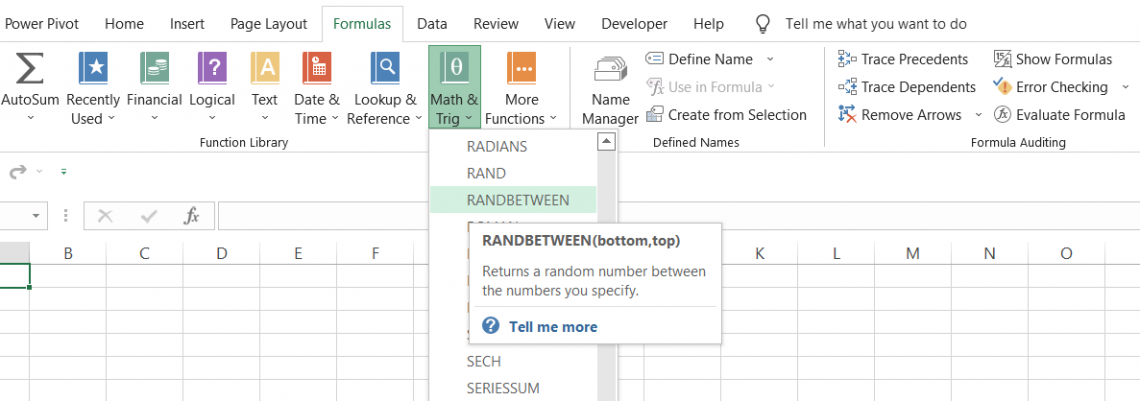An important thing to remember is that the function is very volatile - meaning every time you open the workbook, the value returned using the function will change.

The value will also change if you have enabled the option to auto-calculate formulas in Excel or press F9 to refresh the spreadsheet.

The function can be used to generate a random dataset between constraints that can be set (for example, the weight of a person)

The syntax for the function is

=RANDBETWEEN(bottom, top)

where,

bottom = (required) smallest integer that will be returned by the function

top = (required) largest integer that will be returned by the function

## Examples

The function has a very limited application in financial analysis. However, you can still use it to perform different tasks.Suppose you need to create a height dataset for a group of 50 students. Individually assigning a random integer can take a lot of your time, which, in this fast-paced world, is not a good thing.

Instead, you can use the function and assign a lower and upper limit for the height. The function will then automatically return a set of random numbers for the heights of each of the students.

In financial analysis, if you are unsure as to what a specific assumption should be made for, let's say, the revenue at an organization, you can assume a random number based on the industry-specific standard of the upper and lower limits of related organizations.

If you are creative enough, which you absolutely are, you will come up with different situations where you can add in various other Excel functions.

Some of these situations are shown below:

### Example #1

Suppose you need to create a random dataset for marks scored by students in their exams. We will assume that the minimum mark scored by the students is 35 while the maximum mark scored is 90. The spreadsheet is illustrated below: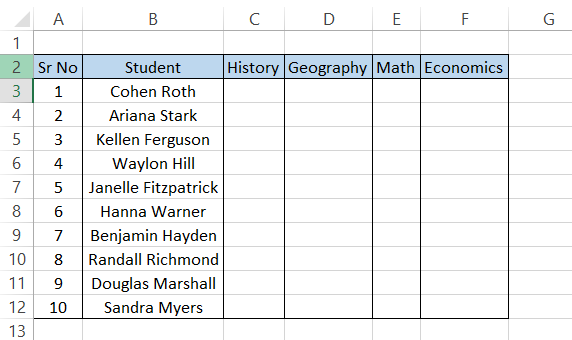You will use the formula =RANDBETWEEN(35,90) in the entire range (i.e. C3:F12), giving you the following random numbers in the spreadsheet: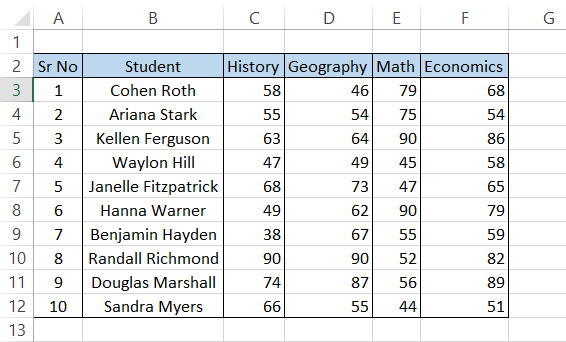If you observe, you will see that the function does not return unique random numbers in the range where the formula is used. In cells C10 and D10, both cells have the marks scored by 'Randall Richmond' as 90.

While nothing is wrong with the function being unable to return unique random numbers (as that makes it truly random), it may be considered a drawback in some situations.

### Example #2

The special thing about this function is that you can also return random letters using a combination of the CHAR, RANDBETWEEN, and CODE functions in Excel.

The CHAR function returns a character based on the number input inside the parenthesis. For example, CHAR(65) will return 'A' (capitalized).

The numbers 65 to 90 represent the alphabet, from A - Z, while anything before 65 represents other characters that can be used in Excel.

The CODE function returns ANSI character codes based on the character you input in the parenthesis. For example, if you use the formula =CODE("A"), it will return the result 65, which is equal to our letter A.

If you are well aware of the ANSI character codes chart, you can directly use the formula =CHAR(RANDBETWEEN(65,90)). It will randomly return letters between A (65) and Z (90).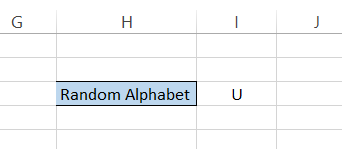However, it won't always be the case that you are familiar with the code needed to return a certain character. For example, can you return the letters between the lowercase' a and lowercase 'z' without looking at the ANSI character code chart?

In this case, you will use the formula =CHAR(RANDBETWEEN(CODE("a"),CODE("z" ))), which will randomly give you a lowercase letter between a and z: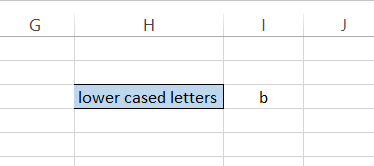If you want to generate random passwords that consist of capitalized letters followed by lowercase letters, a number, and a special character at the end, you will use the following formula:

=CHAR(RANDBETWEEN(CODE("A"),CODE("Z")))&CHAR(RANDBETWEEN(CODE("a"),CODE("z")))&CHAR(RANDBETWEEN(CODE("0"),CODE("9")))&CHAR(RANDBETWEEN(CODE("!"),CODE("/"))), giving us the following result: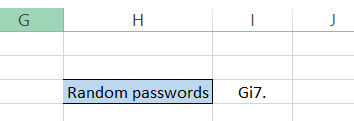Concatenating the function can give you more complex random values than just using a single function.

Note

Check what the code for a specific character is in the ANSI character code chart to get the best result in the spreadsheet.

### Example #3

If you can return random characters using the function, you can generate random dates as well. For example, let's say you are planning to go on a road trip with your friends but can't seem to decide on a date.

By mutual agreement, you all decide that you can start the trip anytime between July 5th, 2022, and August 10th, 2022.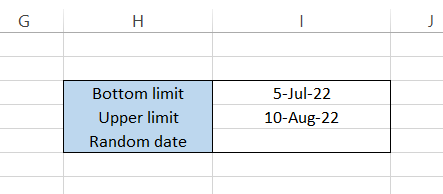You will use the formula =RANDBETWEEN(DATEVALUE("5-Jul-22"),DATEVALUE("10-Aug-2022")), which will return the following: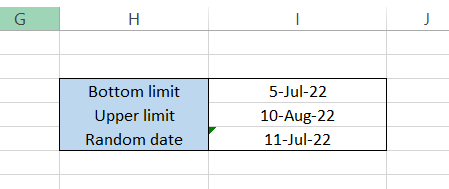You need to be extremely careful with the formatting, or else you won't get the desired result. If you want to avoid formatting entirely, you can also use the DATE function instead of the DATEVALUE function.

The DATE function takes in the argument for the day, month and year separately in the form of numbers, eliminating any chance of errors.

The formula using the DATE function becomes =RANDBETWEEN(DATE(2022,7,5),DATE(2022,8,10)), which will give you the following result: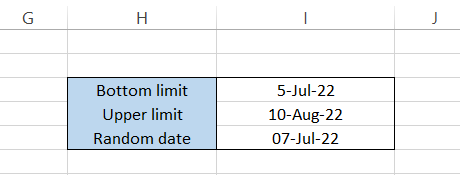So, it seems like, either way, the best date for a road trip is around the 7th to 11th of July, 2022. Pack up your bags and head out if the trip isn't already planned! We have heard that the Phoenix to Page Arizona road trip is absolute beauty all through the year.Begin your journey into Excel modeling with our free Excel Modeling Crash Course.

## RANDBETWEEN Function vs. RAND function

Similar to the function being discussed, the RAND function also generates random numbers. However, it is only limited to returning numbers between 0 and 1. The syntax for the RAND function is:

=RAND()

It does not take any argument inside the parenthesis (the round brackets). So, if you use the formula =RAND() in the spreadsheet, expect a number like 0.687949.

Yes, it will sometimes generate that many digits after the decimal point. You can, however, limit this using the ROUND function.

You can also specify an upper and lower limit and return random numbers, which we have covered in our dedicated article on the RAND function. However, we still advise that the best function to return random numbers between a user-defined range is RANDBETWEEN.

The only upper hand that the RAND function has is that it can also generate decimal numbers (for example, height in feet or weight in kg), while the latter function can only return random integers.

In case you need to return random decimal numbers using the RANDBETWEEN function, say between 20 and 40, you will use =RANDBETWEEN(20,40)*RAND(), returning the following result: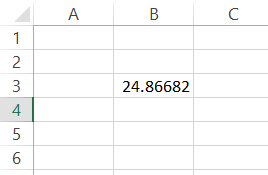So, multiplying the result with the RAND() function actually doesn't give the most accurate results, but it fulfills the purpose of returning decimal numbers. We will still advise using the dedicated method that involves the RAND() function.

Key Takeways

• Being a volatile function, every time you open the spreadsheet or perform calculations in Excel, RANDBETWEEN will return a different random number within the user-defined range.
• Since the function keeps on performing automatic calculations, it slows down the processing speed of Excel.
• If you don't want the result to change, you can press Ctrl + C to copy the result, then Ctrl + Alt + V, and then select 'Values' in the Paste Special dialog box to hard code the result.### Everything You Need To Master Excel Modeling

To Help You Thrive in the Most Prestigious Jobs on Wall Street.

Researched and Authored by Akash Bagul | Linkedin

Reviewed and edited by James Fazeli-Sinaki | LinkedIn